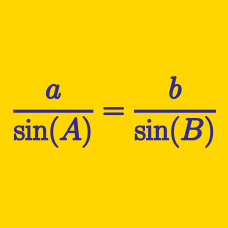Geometry

# Cosine Rule

If triangle $ABC$ has side lengths $a=7$, $b=12$ and $c=11$, the value of $\cos A$ can be expressed as $\frac{p}{q}$, where $p$ and $q$ are coprime positive integers. What is the value of $p+q$?

Details and assumptions

$a$, $b$ and $c$ are the lengths of the sides opposite to the vertices $A$, $B$ and $C$, respectively.

In a triangle, an angle of $60^\circ$ is formed by two sides of lengths $4$ and $11$. If the length of the remaining side is $\sqrt{a}$, what is $a$?

If parallelogram $ABCD$ has $\overline{AB}=8$, $\overline{BC}=4$ and $\angle ABC=60^{\circ}$, what is the square of the length of the diagonal $\overline{BD}$?

Let $ABC$ be a triangle such that $\angle A = 30^{\circ}$, $a = 9 \sqrt{3}$ and $c = 18 \sqrt{3}$. What is the value of $b$?

Details and assumptions

$a$, $b$ and $c$ are the lengths of the sides opposite to the vertices $A$, $B$ and $C$, respectively.

If triangle $ABC$ has $\angle A = 45^{\circ}$, $b=28$ and $c=\sqrt{6}+14\sqrt{2}$, what is the value of $a^2$?

Details and assumptions

$a$, $b$ and $c$ are the lengths of the sides opposite to the vertices $A$, $B$ and $C$, respectively.

×